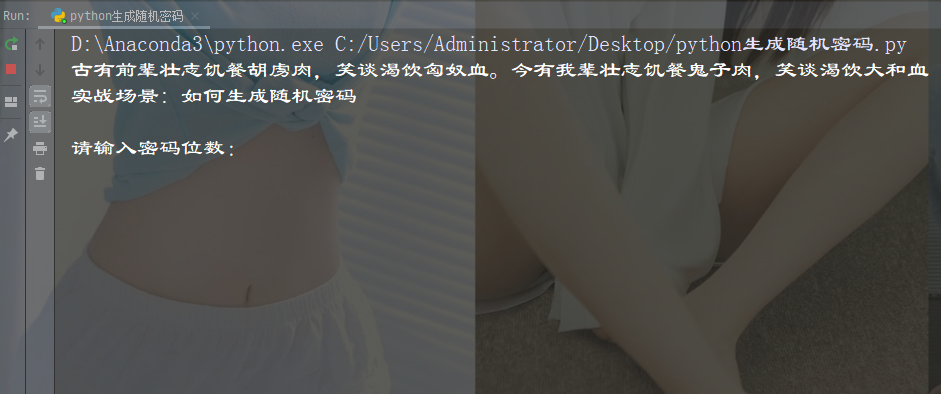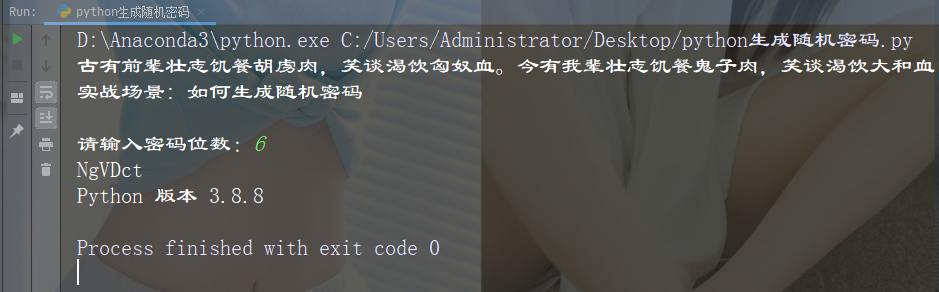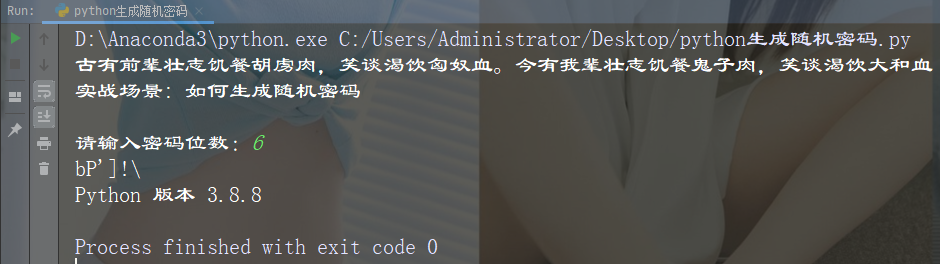• 文件读写
• 基础语法
• 字符串处理
• 字符拼接

```import platform
import string
import random

# 我还给大家准备了这些资料：Python视频教程、100本Python电子书、基础、爬虫、数据分析、web开发、机器学习、人工智能、面试题、Python学习路线图、问题解答！
# 都放在这个扣群啦：279199867```

```words = string.ascii_lowercase + string.ascii_uppercase + string.digits + string.punctuation

len = int(input("请输入密码位数："))```

`chosen = random.sample(words, len)`

`password = "".join(chosen)`

• 小写字母：string.ascii_lowercase；
• 大写字母：string.ascii_uppercase；
• 数字：string.digits；
• 标点符号：string.punctuation

```import platform
import string
import random

print("古有前辈壮志饥餐胡虏肉，笑谈渴饮匈奴血。今有我辈壮志饥餐鬼子肉，笑谈渴饮大和血")
print("实战场景: 如何生成随机密码 \n")

words = string.ascii_lowercase + string.ascii_uppercase + string.digits + string.punctuation

len = int(input("请输入密码位数："))

chosen = random.sample(words, len)

print("Python 版本", platform.python_version())```